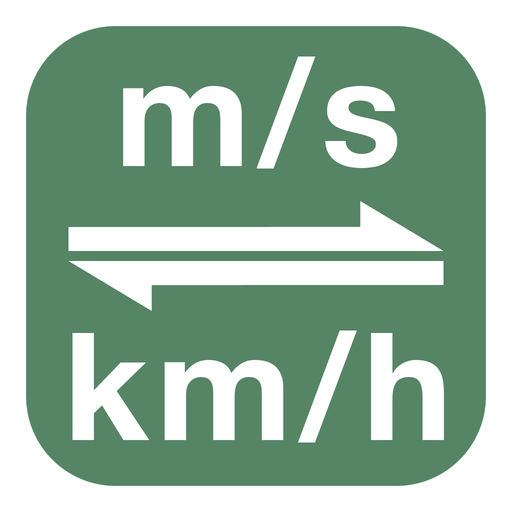Meter Per Second To Kilometer Per Hour | m/s to km/h
Removed from the App Store# Meter Per Second To Kilometer Per Hour | m/s to km/h

## Meta Technologies GmbH

\$0.99
0

0 Ratings

Release Date

2016-06-30

Size

10.5 MB

### Screenshots for iPhone

iPhone
Meter Per Second To Kilometer Per Hour | m/s to km/h Description
Quickest and easiest way to convert between meter per second and kilometer per hour.

Features:
- Easy to use
- Convert in both directions
- No InAppPurchases

Alternative spelling:
- convert meter per second to kilometer per hour
- convert meters per second to kilometer per hour
- convert meter per second to kilometers per hour
- convert meters per second to kilometers per hour
- convert m/s to kilometer per hour
- convert m/s to kilometers per hour
- convert meter per second to km/h
- convert meters per second to km/h
- convert m/s to km/h
- convert meter per second to kilometre per hour
- convert meters per second to kilometre per hour
- convert meter per second to kilometres per hour
- convert meters per second to kilometres per hour
- convert m/s to kilometre per hour
- convert m/s to kilometres per hour
- convert meter per second in kilometer per hour
- convert meters per second in kilometer per hour
- convert meter per second in kilometers per hour
- convert meters per second in kilometers per hour
- convert m/s in kilometer per hour
- convert m/s in kilometers per hour
- convert meter per second in km/h
- convert meters per second in km/h
- convert m/s in km/h
- convert meter per second in kilometre per hour
- convert meters per second in kilometre per hour
- convert meter per second in kilometres per hour
- convert meters per second in kilometres per hour
- convert m/s in kilometre per hour
- convert m/s in kilometres per hour
Meter Per Second To Kilometer Per Hour | m/s to km/h 2.0.0 Update
2016-06-30 Version History
More